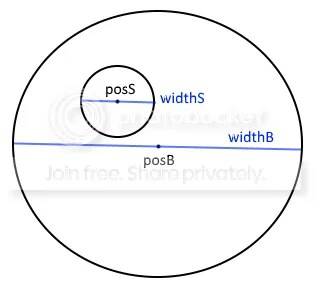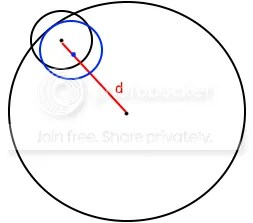I was helping someone on the Codea forum with a simple joystick problem, and I thought I’d share it with you and use it to help explain the math, which really isn’t that complex.

This is the scene.

1. We have a joystick which is a big circle with a small circle inside.
2. You move the small circle round with your finger to steer something on the screen
3. The small circle can’t move outside the big circle
4. When you take your finger off the screen, the small circle goes back to the centre of the big circle

Let’s make up some variable names

• posB = screen position of the centre of the big circle
• posS = screen position of the centre of the small circle
• widthB = diameter of the big circle
• widthS = diameter of the small circleWe need to make sure posS is not further than (widthB – widthS)/2 pixels from posB. How do I get this result?

The radii of the two circles are widthB/2 and widthS/2. To make sure the small circle is totally inside the big circle, its centre position needs to be widthS/2 pixels from the edge – the red line shown below – which is widthB/2 – widthS/2 pixels from the centre of the big circle.We could control the position of the small circle with a lot of if tests that check x and y positions, but I want to show you a more elegant method and explain some useful graphics math.

So here is my touched function that will move the small circle around inside the big circle. I’ve numbered each line so I can explain it below.

```1 function JoystickTouched(touch)
2    if touch.stat==ENDED then posS = posB
3    else
4        t = vec2(touch.x,touch.y)
5        local d=t:dist(posB)
6        if d<(widthB-widthS)/2 then posS=t
7        else posS=posB+(t-posB)/d*(widthB-widthS)/2 end
8    end
9 end
```

 We start with the touch details from the main touched function

 if the player isn’t touching the screen, put the small circle in the middle of the big circle

 otherwise..

 put the touch details in a vec2 for convenience

 calculate the distance between the touch point and the centre of the large circle

 if the distance is less than (widthB – widthS)/2 , we can put the centre of the small circle at the touch point

 but if the distance is greater, the small circle won’t fit inside the big circle, and we need to adjust the touch position.

The picture below may help. The small black circle is centred on the touch point, and it is partly outside the big circle. We know this because the distance d (red line below) between the touch point and the centre of the large circle is too large.

We need to slide the touch point closer to the centre of the big circle, along the red line, until all of the small circle is inside the big circle, as shown by the blue circle below. This keeps the joystick pointing in exactly the same direction as the touch, which is what we want.But how do you slide the touch position along the red line to exactly the right place?

First, we can write the touch point t like this: t = posB + v

where v is just the vector needed to get from posB to t.

For example, if posB=vec2(300, 200) and t=vec2(290, 215), then

v = t – posB = vec2(-10, 15)

since  vec2(290, 215) = vec2(300, 200) + vec2(-10, 15)

The length of v is the distance between the two points, or t:dist(posB) = 18, which is the same as sqrt(v.x^2 + v.y^2), which is simple trigonometry, as you will see if you draw a picture of it.

I called this length d in the code above. At the moment, d is too long, and it needs to be made shorter so its length is exactly (widthB – widthS)/2, which means the little circle will just touch the edge of the big circle without going outside it.

We can pro rate our vector v to get the right position along the line, like this

```v2 = v * (widthB - widthS)/2
d
```

So we divide v by the current length d and then multiply it by the length we want. If we add the result v2 to posB, it will give us the exact position we need.

Let’s use the same example as above, where the length was 18, and assume we want a length of 12. The calculation is

```posB = vec2(300, 200)
t = vec2(290, 215)
v = t - posB            =(-10, 15)
d = t:dist(posB)        =18
v2 = v / d * (widthB - widthS)/2 =(-10,15) /18 * 12 = (-6.7,10)
posS = posB + v2        =(300,200) + (-6.7,10) = (293.3,210)
```

And this is exactly what I’ve done in my function above.

### More on vectors

If you want to do graphics programming, you really need to understand vectors. I’ve written about them here. You should also read the Codea reference, which has a lot of functions especially for vectors.

There are also some very useful functions for graphics, especially dot products and cross products.

From → Games, Graphics

One Comment